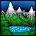# Accelerometer Axis Misalignment Compensation

cancel
Showing results for
Did you mean:

## Accelerometer Axis Misalignment Compensation

1,512 ViewsContributor I

How do you do it and does anyone have a little C/C++ to help or even VB that I could convert.

Many thanks

4 Replies
671 ViewsContributor I

Here's code I used.  Make sure that you have the Z-axis oriented so that it is vertical or experiencing 1G since the calibration works best this way.

void CMMA7455::CalibrateSensor()
{

int err;

m_I2C.ModuleWriteByte(GSENSOR,MCTL_REGISTER,MCTL_MODE0+MCTL_GLVL0);
int x,y,z;
long xAvg,yAvg,zAvg;
xAvg=yAvg=zAvg=0;

int lastXOffset=0;
int lastYOffset=0;
int lastZOffset=0;

SetXAxisOffset(lastXOffset);  TRACE(_T("Last X Offset= %i\n"),lastXOffset);
SetYAxisOffset(lastYOffset);  TRACE(_T("Last Y Offset= %i\n"),lastYOffset);
SetZAxisOffset(lastZOffset);  TRACE(_T("Last Z Offset= %i\n"),lastZOffset);

int loop=0;
do
{
xAvg=yAvg=zAvg=0;
// Read the axes multiple times and average;
{
signed int data;
}
x=int(xAvg>>6);
y=int(yAvg>>6);
z=int(zAvg>>6);
// Calculate the offset.
lastXOffset=lastXOffset+(x*-2);
lastYOffset=lastYOffset+(y*-2);
lastZOffset=lastZOffset+((64-z)*2);

//Set the offset.
SetXAxisOffset(lastXOffset);  TRACE(_T("Last X Offset= %i\n"),lastXOffset);
SetYAxisOffset(lastYOffset);  TRACE(_T("Last Y Offset= %i\n"),lastYOffset);
SetZAxisOffset(lastZOffset);  TRACE(_T("Last Z Offset= %i\n"),lastZOffset);

// Remeasure the values
xAvg=yAvg=zAvg=0;
{
signed int data;
}
x=int(xAvg>>6);
y=int(yAvg>>6);
z=int(zAvg>>6);
loop++;
}
while( ((abs(x)>2) ||(abs(y)>2)||(abs(z-64)>2))&& (loop<10) ) ;

}

I'll leave the other functions as an exercise for the reader. I assume you can already read and write registers on the device.

671 ViewsContributor I

Hello,

I understand the code but why are you doing it?

Many thanks

671 ViewsContributor I

Because like all mechanical devices there are tolerances and variations. If you start up the device and read the values you will usually see that there is some measured deflection on x and y and also that z is greater than or equal to 1g.  Assuming you are using this in a stationary position on earth then all three axes should add up to give a 1g vector. If you arrange the device so that the z-axis is perpendicular to the earths surface, you should see 1g on that axis.

See App note,.AN3745

http://www.freescale.com/files/sensors/doc/app_note/AN3745.pdf

Peter

671 ViewsContributor I

Hello Peter and many thanks for the post.

Mmmm interesting way of doing this offset and scaling. I get the min & max values then use linear interpolation.

However the problem I am trying to find a solution to is compensating for axis alignment errors.

Have found this but math a bit heaving going for me.

http://www.summitinstruments.com/knowledge_center/pdf/TN413.pdf